# Tag Archives: Difficulty 7

## Reachability for 1-Conservative Petri Nets

As promised, here is the next problem, also using Petri nets.  I’m not 100% convinced that my definition of “1-Conservative” is accurate, but it seems to work for this reduction.

The problem: Reachability for 1-Conservative Petri nets.  This is problem MS4 in the appendix.

The description: A “1-Conservative” Petri net has each transition preserve the number of tokens on each side of the transition, and has at most 1 token per edge.  Given such a Petri net and a state M, is there a sequence of transitions that can reach M from the starting state?

Example: I had a hard time finding a non-trivial example of a 1-conservative network.  But maybe that’s ok.  Here’s another figure from the Murata paper: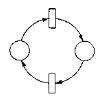There are no tokens in this picture.  But notice that if we start with no tokens, no state with tokens is reachable.  If we start with a token in the left state, then both 1-token configurations are reachable, but we won’t be able to reach a configuration with 0 tokens or 2 tokens in it.

Reduction: This is Theorem 3.1 in the Jones, Landweber, and Lien paper.  The reduction is from Linear Bounded Automaton Acceptance.

So we start with a LBA M, with p tape symbols, and m states.  It has an input string $x1x2..xn$.  Our task is to build a 1-Conservative Petri Net.

The first set of places in the Petri net will be represented by Aij,  0 <= i <= n+1, 1 <= j <= p.  This will represent the tape.  Aij will have a token iff space i of the tape has symbol j.

We will also have places labeled Qij, 0 <= i <= n+1, 1 <= j <= m.  This will simulate the states.  Qij will have a token iff the head is over space i of the tape while the machine is in state j.

We’ll also have 2 special places C and D.  Tokens start in all of the Aij places corresponding to the input string of the tape, and in Q11 (the machine starts in state 1 over cell 1 of the tape).

The transitions in the net are based on the transitions in the machine.  If we have a transition from state qs and symbol at to state qr, writing a symbol al we add a transition Qis + Ait -> Qjr + Ail for all i.  “j” in the destination is either i, i+1, or i-1, depending on if the head stays, moves right, or moves left.

If we are in a final state qs, we add a transition Qis->C for all i.

We also add the transition C+Aij -> C+D.

Note that all transitions have 1 token per edge and preserve the number of tokens, so this is a 1-Conservative Petri net.

Our destination state is:

• 1 token in C
• N tokens in D
• 1 token in each Ai1

Since all of these transitions simulate the movement in the LBA, if the LBA accepts the string, we follow all of the transitions to eventually put the token in C.  This allows us to do the last transition to put the tokens in D.

In the other direction, if we can reach the desired state, we must have put a token in C.  To get there, we must have gotten to a final state qf.  Thus, the LBA must have accepted the string (by following the transitions we took in the Petri Net to get to that qf state).

Difficulty:  7.  I like how they can represent all combinations of tape symbols and locations as places.  I’m a little worried because it looks like this assumes you’ll never go beyond the end of the input string, where I thought an LBA was allowed to use a linear multiple of the input string’s size.  Is there some kind of equivalence proof that says an LBA can only need its own input string’s space exactly?

## Betweenness

The last section is on “Miscellaneous” problems.  There are some weird ones in here, but we start with a pretty straightforward number problem.

The problem: Betweenness.  This is problem MS1 in the appendix.

The description: Given a set C of triples (a,b,c), where each element of each triple comes from a superset A, can we find an ordering of the elements of A such that according to that ordering, each triple is in either increasing or decreasing order?

Example: It’s actually hard to do a good example for this because most groups of symbols (digits, letters, subscripts, …) have an implicit ordering of elements, and to go against that would be confusing.  Having random symbols without connotations of meaning gets pretty confusing pretty quickly as well.

So let’s lean in and say A = {a,b,c,d,e,f} and our triples are:

(a,d,f)
(f,d,b)
(h,d,a)
(f,g,h)
(d,f,h)

We’re allowed any ordering of the elements of A, and the “normal” lexicographical one with ‘a’ first and ‘f’ last satisfies the betweenness rules.

But now suppose we add the new triple: (b,a,d).  That is an illegal triple in our normal order, but if we change our ordering so that ‘b’ is first and ‘a’ is second, we can still satisfy all of the triples.

Reduction: The paper by Opatrny uses Hypergraph 2-Colorability (which we called “Set Splitting”).  Recall that our reduction only made sets of size 3, so we can start with an instance where each set has at most 3 elements (or, each hyperedge connects at most 3 vertices).

So, suppose we have a set of N vertices s1..sn, a set of J 3-vertex hyperedges (ai, bi, ci), as well as M 2-vertex “regular” edges (di, ei).

Our first job is to build superset A.  It has:

• One symbol for each vertex,
• A new symbol X.
• A set of J new symbols Yk, one for each 3-vertex hyperedge.

Now we build C.

• Each hyperedge (ak, bk, ck) generates two triples: (ak, Yk, bk) and (Yk, X, ck)
• Each two-vertex edge (dk, ek) generates the triple (dk, X, ek)

Suppose the graph had a 2-coloring.  Recall that meant that each vertex had a color (the paper uses red and blue) so that each edge and hyperedge touches vertices of both colors.  We need to make an ordering of A that satisfies all triples.  The ordering will have positive,  negative, and rational ranks.

• X has rank of 0.
• If vertex si is red, its rank is i.  If it’s blue, its rank is -i.
• Each Yi was associated with a hyperedge (ai, bi, ci).
• If ai and bi have the same color (and so the same sign), Yi‘s rank is min(rank(ai), rank(bi)) +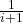.
• If they have different colors, the rank isif ci is blue, and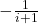if ci is red

So, suppose every edge had 2 colors.  We know:

• If ai and bi are the same color, they are the same sign, and Yi is between them, satisfying the (ak, Yk, bk) triple.
• If ai and bi are the same color, ci must be the other color (and the other sign from Yi), satisfying the (Yk, X, ck) triple.
• If ai and bi are different colors, they are different signs.  Setting Yi to a value between 1 and -1 satisfies the (ak, Yk, bk) triple.
• If ai and bi are different colors, ci can be either color, so making Yi the opposite sign as ci satisfies the (Yk, X, ck) triple.
• An edge with just 2 vertices needs both vertices opposite colors, and thus opposite signs, so the (dk, X, ek) triple is satisfied.

So far, so good.  In the opposite direction, suppose we have a solution to the betweenness instance.  We’ll assign colors to vertices such that a vertex’s color is red if its rank is > X’s rank.  Otherwise, it will be assigned blue.  Our triples will now allow any set of ai, bi, and ci to all be greater than or less than X (or for both di and ei to be greater than or less than X), which means that every edge in the hypergraph has both a red and a blue vertex.

Difficulty: 7.  It’s relatively easy to see what you need to do.  It’s harder to make the numbers actually work.  Even this paper has to resort to using rational numbers for the ranks, which is a fixable problem, but certainly not obvious.

Here is another scheme problem with a different kind of a reduction.

The problem: Non-Containment for Free B-Schemes. This is problem PO18 in the appendix.

The description: A “B-Scheme is” a rooted, directed, acyclic graph where each vertex has outdegree of either 0 (a “leaf”) or 2 (a “test”).  The test vertices label their out edges as “T” or “F”.  Each vertex gets a label.  Test vertices are labeled as boolean Boolean variables, leaves are labeled with function symbols (with the special symbol “Ω” meaning “undefined function”.

Multiple vertices can have the same label, but in a “free” B-scheme, no path from the root to a leaf hits two vertices with the same label.  We can “assign” truth values to the boolean variables, which then define a path through the scheme to a leaf.  The value of the truth assignment is the label given to the leaf we end up in.

Suppse we have two such B-Schemes, S1 and S2.  S1 is contained in S2 if for all assignments of variables, either S1 ends up in Ω, or S1 ends up in a leaf that is the same label as S2‘s leaf with the same assignment.

So, our question is: Given two free B-Schemes S1 and S2, is S1 not  contained in S2?

Example:

Here is our S1: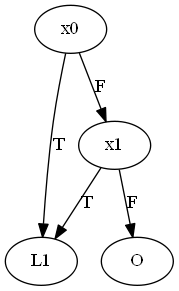“O” is the label for the Ω leaf

Here is S2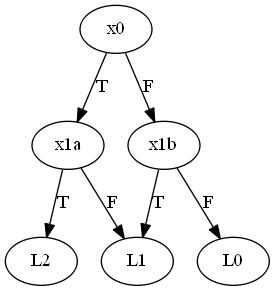(x1a and x1b both are labeled for the variable x1.  I had to give them different names so Graphviz didn’t think they were the same variable.)

S2 returns the number of true assignments of x0 and x1.  S1 correctly finds the same leaf if at least one variable is true, but goes to O otherwise.

But, S1 is NOT contained in S2 because assigning both variables to true puts S1 in L1 and S2 in L2.

Reduction: The paper by Fortune, Hopcroft, and Schmidt defines this problem and reduces from 3SAT. First, we replace all positive occurrences of the same variable xi with new variables ui1..uip (if it occurred p times).  We similarly replace all negative occurrences of xi with vi1..viq (if it appeared negated q times).  Our job is to build two schemes.

S1‘s job is to tell us if the formula is satisfiable.  Each clause (a1,b1, c1), gets a chain of vertices. It goes “across” on F, and down to the first variable of the second clause on T.  If all literals get assigned F, we end up in a Ω leaf.  The final clause has edges from T to our output “f” leaf (Yes, that means the vertex we reach when the formula is satisfiable and all of the clauses are true is labeled “f”.  I’m sorry.)  Here is the paper’s example.  Keep in mind that each of the a,b, and c vertices here represent literals whose actual value in the formula is one of the u and v variables we created above.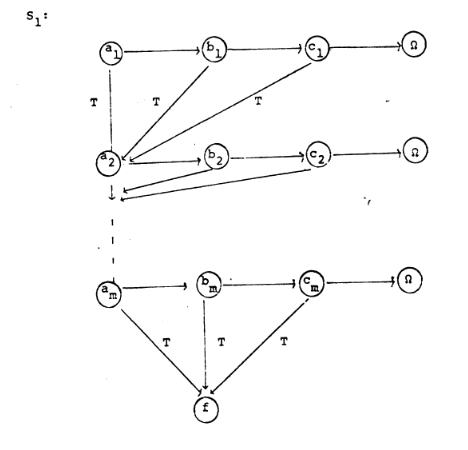S2‘s job is to make sure that all of our new u and v vertices have the same (and consistent) truth values.  If we ever have a ui with a different truth value than some other uj (or if it has the same truth value as its corresponding vj) we jump to f.  If everything is ok, we jump to a new end point g.  Here is the diagram from the paper.  Notice that they are doing this for all of the replacements for all of the variables in one scheme.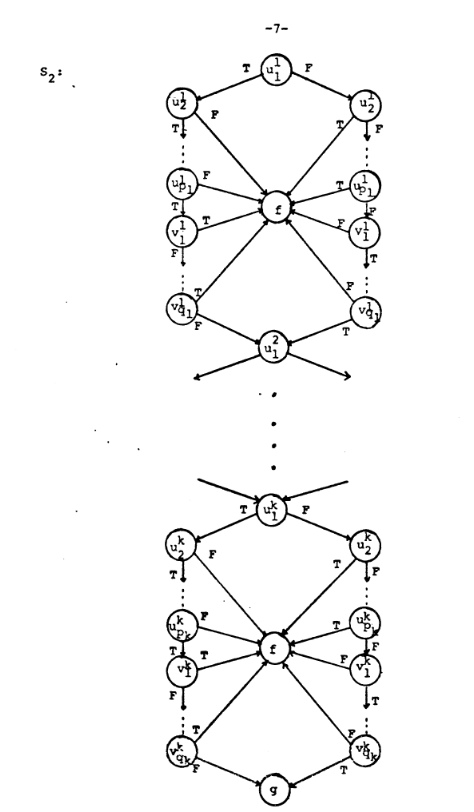If our original formula was satisfiable, we would find a way to make S1 go to f, and since all of the variable assignments are consistent, we could use those assignments to make S2 go to g, so S1 is not contained in S2,  In the other direction, if S1 is not contained in S2, then since all S1 can reach is Ω and f, it must be the case that there is an assignment that leads S1 to f and S2 to g.  S2 ends in g only when its u and v variables are assigned consistently.  S1 goes to f only when its clauses are all satisfied.  Thus, we have found a way to satisfy the formula.

Difficulty: 7.  This is different from the other scheme problems we have seen, where the second scheme is the trivial “yes” scheme.  Here, we need to use both schemes to check different aspects of the solution.  That’s pretty cool.